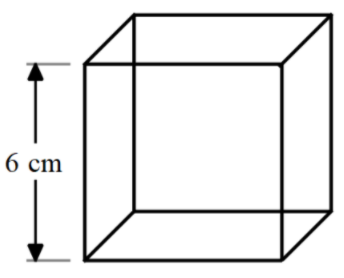Courses
Courses for Kids
Free study material
Free LIVE classes
MoreLIVE
Join Vedantu’s FREE Mastercalss

# Find the volume of a cube of edge 6cm.A). 64${cm}^{3}$B). 125${cm}^{3}$C). 216${cm}^{3}$D). 343${cm}^{3}$Verified
334.5k+ views
Hint: In order to solve this problem, we need to find the formula for the volume of the cube. The formula for the volume of the cube is given by $\text{Volume =}{{\left( \text{side} \right)}^{\text{3}}}$ , where the side is the side of the cube. We just need to substitute the value of the side of the cube and we can get the volume of the cube.

Complete step-by-step solution:
We are given the side of the cube.
The cube is the shape having all the sides equal.
The cube is the 3-D shape made up of 6 squares acting like the faces of the cube.
All the squares are exactly similar and with the side of the same length.
The side of the cube is 6 cm.
It can be shown as follows,The six faces are as follows, front, back, left, right, top, bottom.
Also, we can see that each side of the cube is square.
The volume of the cube is given by,
$\text{Volume = length }\!\!\times\!\!\text{ breadth }\!\!\times\!\!\text{ height}$
We have already seen that all the sides are the same. So, we can modify the formula as follows,
\begin{align} & \text{Volume = side }\!\!\times\!\!\text{ side }\!\!\times\!\!\text{ side} \\ & \text{=}{{\left( \text{side} \right)}^{\text{3}}} \end{align}
Substituting the values, we get,
\begin{align} & \text{Volume}={{\left( 6 \right)}^{3}} \\ & =216 \end{align}
Hence, the volume of the cube is 216 $c{{m}^{3}}$ .

Note: We can straight away give the answers as we can see that all the options the perfect cubes of all the numbers from 4,5,6 and 7. We have been given the edge to be 6. So, the cube of 6 cm so the volume is the cube 6 which is 216 $c{{m}^{3}}$.

Last updated date: 21st Sep 2023
Total views: 334.5k
Views today: 9.34k